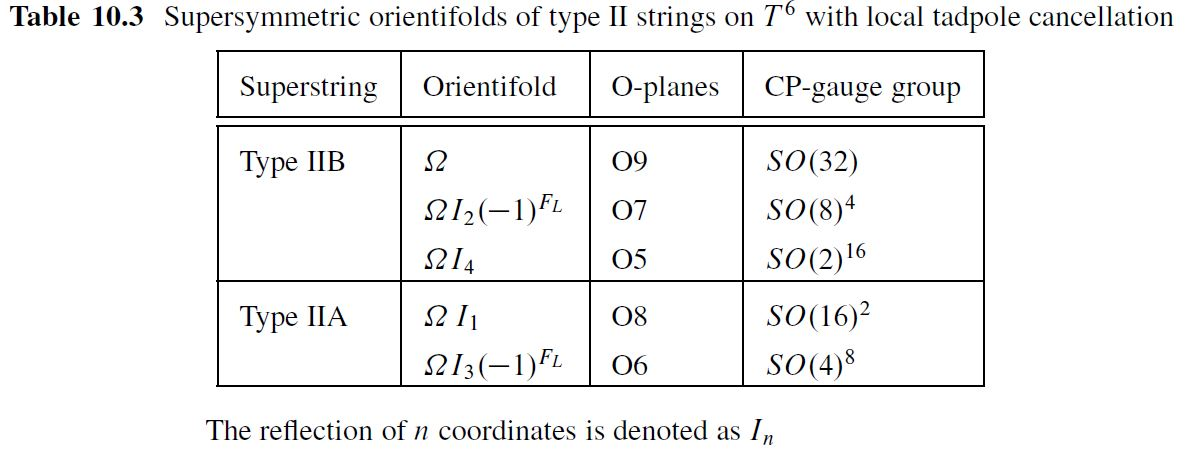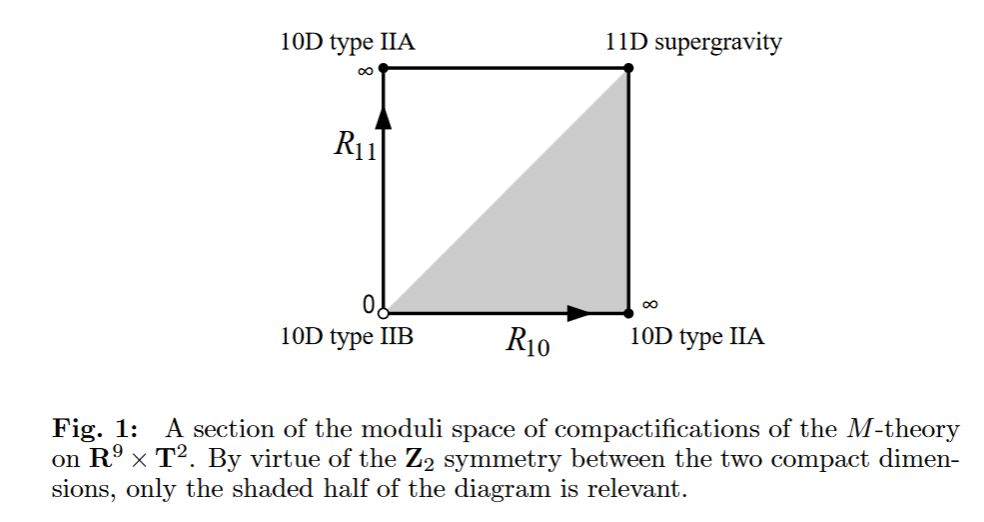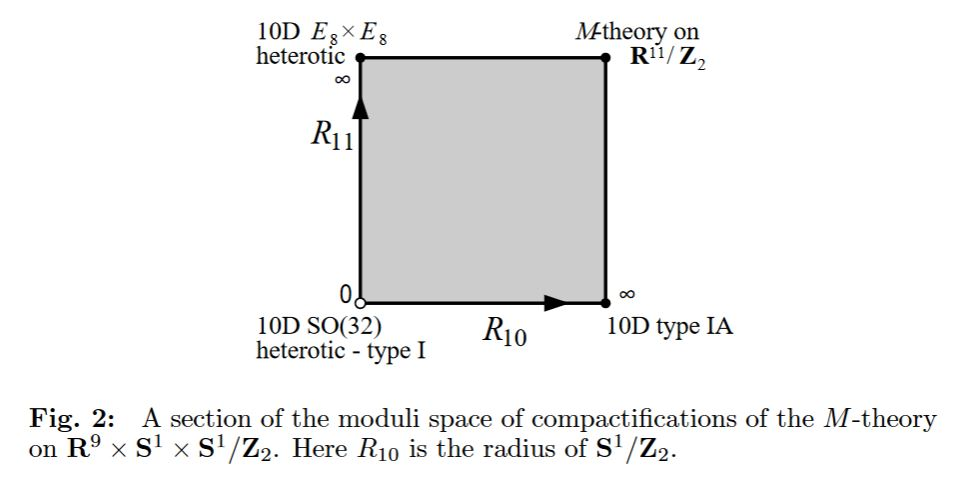# nLab type I string theory

Contents

## Surveys, textbooks and lecture notes

#### Gravity

gravity, supergravity

## Quantum theory

#### Quantum field theory

functorial quantum field theory

## Spin geometry

spin geometry

Dynkin labelsp. orth. groupspin grouppin groupsemi-spin group
SO(2)Spin(2)Pin(2)
B1SO(3)Spin(3)Pin(3)
D2SO(4)Spin(4)Pin(4)
B2SO(5)Spin(5)Pin(5)
D3SO(6)Spin(6)
B3SO(7)Spin(7)
D4SO(8)Spin(8)SO(8)
B4SO(9)Spin(9)
D5SO(10)Spin(10)
B5SO(11)Spin(11)
D6SO(12)Spin(12)
$\vdots$$\vdots$
D8SO(16)Spin(16)SemiSpin(16)
$\vdots$$\vdots$
D16SO(32)Spin(32)SemiSpin(32)

string geometry

# Contents

## Idea

What is called type I string theory is type IIB string theory on orientifold spacetimes, hence on O9-planes.

Its T-dual, called type I’ string theory, is type IIA string theory on O8-planes, which under the duality between M-theory and type IIA string theory is M-theory KK-compactified on the orientifold $S^1 \times S^1 \sslash \mathbb{Z}_2$ (see also M-theory on S1/G_HW times H/G_ADE):

$\array{ M \\ {}^{ \mathllap{S^1 \times S^1/\mathbb{Z}_2 }}\big\downarrow \\ I' &\underset{T}{\leftrightarrow}& I }$table from BLT 13

## Properties

### Tadpole cancellation and $SO(32)$-GUT in Type I

For type I string theory on flat (toroidal) target spacetime orientifolds $\mathbb{R}^{9,1}$ (i.e. for type IIB string theory on flat toroidal O9-planes) RR-field tadpole cancellation requires 32 D-branes (see this Remark for counting D-branes in orientifolds) to cancel the O-plane charge of -32 (here).

Under the duality between type I and heterotic string theory this translates to the semi-spin gauge group SemiSpin(32) of heterotic string theory.

Discussion of type-I string phenomenology and grand unified theory based on SO(32) type-I strings: (MMRB 86, Ibanez-Munoz-Rigolin 98, Yamatsu 17).

### Tadpole cancellation and $SO(16) \times SO(16)$-GUT in Type I’

For type I’ string theory on flat (toroidal) target spacetime orientifolds $X^{8,1} \times \mathbb{S}^1/\mathbb{Z}_2$ (i.e. for type IIA string theory on two flat toroidal O8-planes) RR-field tadpole cancellation requires 16 D-branes (see this Remark for counting D-branes in orientifolds) on each of the two O8-planes to cancel the total O-plane charge of $-32 = 2 \cdot (-16)$ (here).

Discussion of Spin(16)-GUT phenomenology:

(…)

### Orbifolds of type I

Type I’ on toroidal orientifolds with ADE-singularities (e.g. Bergman&Rodriguez-Gomez 12, Sec. 3)

(…)

### Dualities

#### Horava-Witten theory

One considers the KK-compactification of M-theory on a Z/2-orbifold of a torus, hence of the Cartesian product of two circles

$\array{ & S^1_A &\times& S^1_B \\ \text{radius}: & R_{11} && R_{10} }$

such that the reduction on the first factor $S^1_A$ corresponds to the duality between M-theory and type IIA string theory, hence so that subsequent T-duality along the second factor yields type IIB string theory (in its F-theory-incarnation). Now the diffeomorphism which exchanges the two circle factors and hence should be a symmetry of M-theory is interpreted as S-duality in type II string theory:

$IIB \overset{S}{\leftrightarrow} IIB$graphics taken from Horava-Witten 95, p. 15

If one considers this situation additionally with a $\mathbb{Z}/2\mathbb{Z}$-orbifold quotient of the first circle factor, one obtains the duality between M-theory and heterotic string theory (Horava-Witten theory). If instead one performs it on the second circle factor, one obtains type I string theory.

Here in both cases the involution action is by reflection of the circle at a line through its center. Hence if we identify $S^1 \simeq \mathbb{R} / \mathbb{Z}$ then the action is by multiplication by /1 on the real line.

In summary:

M-theory on

• $(S^1_A \sslash \mathbb{Z}_2 ) \times S^1_B$ yields heterotic string theory

• $S^1_A \times \left( S^1_B \sslash \mathbb{Z}_2 \right)$ yields type I' string theory

Hence the S-duality that swaps the two circle factors corresponds to a duality between heterotic E and type I’ string theory. And T-dualizing turns this into a duality between type I and heterotic string theory.

$\array{ HE &\overset{KK/\mathbb{Z}^A_2}{\leftrightarrow}& M &\overset{KK/\mathbb{Z}^B_2}{\leftrightarrow}& I' \\ \mathllap{T}\updownarrow && && \updownarrow \mathrlap{T} \\ HO && \underset{\phantom{A}S\phantom{A}}{\leftrightarrow} && I }$graphics taken from Horava-Witten 95, p. 16

cohomology theories of string theory fields on orientifolds

string theoryB-field$B$-field moduliRR-field
bosonic stringline 2-bundleordinary cohomology $H\mathbb{Z}^3$
type II superstringsuper line 2-bundle$Pic(KU)//\mathbb{Z}_2$KR-theory $KR^\bullet$
type IIA superstringsuper line 2-bundle$B GL_1(KU)$KU-theory $KU^1$
type IIB superstringsuper line 2-bundle$B GL_1(KU)$KU-theory $KU^0$
type I superstringsuper line 2-bundle$Pic(KU)//\mathbb{Z}_2$KO-theory $KO$
type $\tilde I$ superstringsuper line 2-bundle$Pic(KU)//\mathbb{Z}_2$KSC-theory $KSC$

### General

Relation to M-theory (via Horava-Witten theory):

A comprehensive discussion of the (differential) cohomological nature of general type II/type I orientifold backgrounds is in

with details in

Related lecture notes / slides include

• Jacques Distler, Orientifolds and Twisted KR-Theory (2008) (pdf)

• Daniel Freed, Dirac charge quantiation, K-theory, and orientifolds, talk at a workshop Mathematical methods in general relativity and quantum field theories, November, 2009 (pdf)

• Greg Moore, The RR-charge of an orientifold, Oberwolfach talk 2010 (pdf, pdf, ppt)

### Type I’

Original articles on type I' string theory:

Type I’ on toroidal orientifolds with ADE-singularities (dual to heterotic M-theory on ADE-orbifolds):

• Oren Bergman, Diego Rodriguez-Gomez, 5d quivers and their $AdS_6$ duals, JHEP07 (2012) 171 (arxiv:1206.3503)

### Phenomenology

Type I string phenomenology and discussion of GUTs based on SO(32) type I strings (see also at heterotic phenomenology):

• H.S. Mani, A. Mukherjee, R. Ramachandran, A.P. Balachandran, Embedding of $SU(5)$ GUT in $SO(32)$ superstring theories, Nuclear Physics B Volume 263, Issues 3–4, 27 January 1986, Pages 621-628 (arXiv:10.1016/0550-3213(86)90277-4)

• Luis Ibáñez, C. Muñoz, S. Rigolin, Aspects of Type I String Phenomenology, Nucl.Phys. B553 (1999) 43-80 (arXiv:hep-ph/9812397)

• Emilian Dudas, Theory and Phenomenology of Type I strings and M-theory, Class. Quant. Grav.17:R41-R116, 2000 (arXiv:hep-ph/0006190)

• Naoki Yamatsu, String-Inspired Special Grand Unification, Progress of Theoretical and Experimental Physics, Volume 2017, Issue 10, 1 (arXiv:1708.02078, doi:10.1093/ptep/ptx135)

### Duality

Discussion of duality with heterotic string theory includes the following.

The original conjecture is due to

More details are then in

### Geometric engineering of $D=6$$\mathcal{N}=(1,0)$ SCFT

On D=6 N=(1,0) SCFTs via geometric engineering on M5-branes/NS5-branes at D-, E-type ADE-singularities, notably from M-theory on S1/G_HW times H/G_ADE, hence from orbifolds of type I' string theory (see at half NS5-brane):